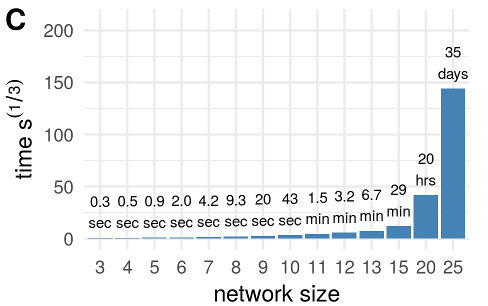# DGM: Dynamical graphical models for multivariate time series data to estimate directed dynamic networks in functional MRI

The aim of this package is to study directed dynamic functional connectivity in fuctional MRI. Dynamic graphical models (DGM) belong to the family of Dynamic Bayesian Networks. DGM is a collection of Dynamic Linear Models (DLM) , a dynamic mutiple regression at each node. Moreover, DGM searches through all possible parent models and provides an interpretable fit in terms of regression model for each network node. There is a special variant of DGM called Multiregression Dyanmic Models (MDM) which constrain the network to a acyclic graph [2,3], but with DGM, we do not use this constrain.

Current research aims to fully characterize DGM using simulations and big data from the Human Connectome Project (HCP) in order to test validity and reliability to make this new method available for neuroimaging research.

## Reference

Schwab, S., Harbord, R., Zerbi, V., Elliott, L., Afyouni, S., Smith, J. Q., … Nichols, T. E. (2017). Directed functional connectivity using dynamic graphical models. bioRxiv. https://doi.org/10.1101/198887.

1. West, M., & Harrison, J. (1997). Bayesian Forecasting and Dynamic Models. Springer New York.
2. Costa, L., Smith, J., Nichols, T., Cussens, J., Duff, E. P., & Makin, T. R. (2015). Searching Multiregression Dynamic Models of Resting-State fMRI Networks Using Integer Programming. Bayesian Analysis , 10(2), 441–478. https://doi.org/10.1214/14-BA913.
3. Queen, C. M., & Smith, J. Q. (1993). Multiregression Dynamic Models. Journal of the Royal Statistical Society. Series B, Statistical Methodology, 55(4), 849–870. Retrieved from http://www.jstor.org/stable/2345998.

## User Guide

### Installation

The installation is 2MB, with dependencies approx. 86MB.

#### From CRAN:

``install.packages("DGM")``

#### Latest develop version

``````install.packages("devtools")
library(devtools)
install_github("schw4b/DGM", ref = "develop")``````

### Running a DGM example with simulated data

We load simulation data of a 5-node network with 200 samples (time points) of one subject. Time series should already be mean centered.

``````library(DGM)
data("utestdata")
dim(myts)
 200   5``````

Now, let’s do a full search across all possible parent models of the size n2(n-1). Here, with n=5, we have 16 possible models for each node, for example for node 3.

``````result=exhaustive.search(myts,3)
result\$model.store
[,1]      [,2]      [,3]      [,4]      [,5]      [,6]     [,7]      [,8]      [,9]     [,10]     [,11]     [,12]     [,13]     [,14]     [,15]     [,16]
[1,]    1.0000    2.0000    3.0000    4.0000    5.0000    6.0000    7.000    8.0000    9.0000   10.0000   11.0000   12.0000   13.0000   14.0000   15.0000   16.0000
[2,]    0.0000    1.0000    2.0000    4.0000    5.0000    1.0000    1.000    1.0000    2.0000    2.0000    4.0000    1.0000    1.0000    1.0000    2.0000    1.0000
[3,]    0.0000    0.0000    0.0000    0.0000    0.0000    2.0000    4.000    5.0000    4.0000    5.0000    5.0000    2.0000    2.0000    4.0000    4.0000    2.0000
[4,]    0.0000    0.0000    0.0000    0.0000    0.0000    0.0000    0.000    0.0000    0.0000    0.0000    0.0000    4.0000    5.0000    5.0000    5.0000    4.0000
[5,]    0.0000    0.0000    0.0000    0.0000    0.0000    0.0000    0.000    0.0000    0.0000    0.0000    0.0000    0.0000    0.0000    0.0000    0.0000    5.0000
[6,] -353.6734 -348.1476 -329.9119 -359.3472 -346.9735 -348.4044 -355.495 -347.4541 -337.3578 -333.6073 -353.8772 -349.6878 -346.9843 -358.2056 -341.7462 -355.5821
[7,]    0.5000    0.7300    0.6800    0.7700    0.7600    0.7800    0.800    0.7900    0.7300    0.7700    0.8100    0.7900    0.8300    0.8400    0.7800    0.8300``````

The columns are the 16 different models. First row indicates model number, rows 2-5 the parents, row 6 the model evidence, a log likelihood, and row 7 the discount factor delta, reflecting the smoothness of the time-varying regression coefficent (theta). To get the winning model, we simply maximaze across model evidence.

``````which.max(result\$model.store[6,])
 3``````

Model number 3 with node 2 as a parent is most likely.

### Analysis on the subject-level

We do a full search on the subject level (exhautive search on each node). The list returned contains all the models (`models`), the winning models (`winner`), the adjacency matrix of the network (`adj`).

``````s=subject(myts)
names(s)

The `adj` structure contains the adjacency matrix of the network (`am`), the model evidence (`lpl`), and the discount factors delta (`df`).

``````names(s\$adj)
 "am"  "lpl" "df"``````

### Plot network as adjacency matrix

The full network structure can be plotted as follows:

``gplotMat(s\$adj\$am, hasColMap = F, title = "network")``
Estimates for a time-series with 1200 samples with a 2.6GHz CPU.